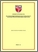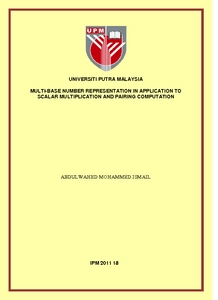# Multi-base number representation in application to scalar multiplication and pairing computation

## Citation

Mohammed Ismail, Abdelwahed (2011) Multi-base number representation in application to scalar multiplication and pairing computation. Doctoral thesis, Universiti Putra Malaysia.

## Abstract

Elliptic curves scalar multiplication over finite fields has become a highly active research area. The efficiency of elliptic curves scalar multiplication is about keeping the memory and running time to be as low as possible. Providing new methods using chains beyond the binary chain will increase the efficiency and speed of elliptic based curve cryptosystems. In this work, an extension of Greedy algorithm called xGreedy, is proposed. It finds the best approximated powers of each base to be used to convert large integers to new addition chains. Then multi-bases number representation is applied to produce new elliptic curve single-scalar multiplication algorithm. Multi-base chains are provided with and without doubling operation. Due to the efficiency of point halving and its low costs,for ordinary curves defined over binary finite fields, the results show fewer arithmetic operations. The multi-bases number representation is also applied to construct another new elliptic curve joint-scalar multiplication algorithm, called the joint-multi base algorithm. This method computes two scalar multiplications simultaneously using multi bases in the chain. The joint-scalar is important in applications related to digital signature verification, such as elliptic curve digital signature algorithm and El Gamal signature scheme. The method is evaluated over different coordinate systems. The results show clear improvement compared to some previous methods proposed for the same purpose. The efficiency of pairing-based cryptosystems depends on the elliptic curves scalar multiplication efficiency. Miller’s algorithm for computing the Tate pairing, originally used the binary chains with corresponding point doubling operation in evaluating the rational function. Multi-bases number representation is used to construct new versions of Miller’s algorithm. These algorithms are formulated for computing Tate and ate pairing. The theoretical calculations and analyses are focused on the Tate pairing. The results show a speeding up. All the above developments are aimed at reducing the running cost of the pairing computation in pairing-based cryptosystems.Preview
PDF
IPM 2011 18R.pdfView Item